# 8-图2¶

  1 2 3 4 5 6 7 8 9 10 11 12 13 14 15 16 17 18 19 20 21 22 ADT 图 Graph Data 顶点的有穷非空集合和边的集合 Operation CreateGraph(*G, V, E): 按照顶点集V和边集E的定义构造图G DestoryGraph(G*): 图G存在则销毁 LocateVex(G*, v): 若图G中存在顶点v，则返回v在图中的顶点数组的下标 GetVex(G*, v): 返回图中顶点v的值 PutVex(G*, v, value): 将图G中顶点v赋值value FirstNeighbor(G*, v): 返回顶点v的第一个邻接点，若无则返回空 NextNeighbor(G*, v1, v2): 返回顶点v1相对于顶点v2的下一个邻接点，若v2是v1的最后一个邻接点则返回空 InsertVex(G*, v): 在图G中增加顶点v DeleteVex(G*, v): 删除图G中顶点v及其相关的边/弧 InsertArc(G*, v1, v2): 在图G中增加弧，若G是无向图，还需添加对称弧 DeleteArc(G*, v1, v2): 在图G中删除弧，若G是无向图，还需删除对称弧 DFSTraverse(G*): 对图G中进行深度优先遍历 BFSTraverse(G*): 对图G中进行广度优先遍历 Adjacent(G*, v1, v2): 判断图G中是否存在边/弧 Neighbors(G*, v): 列出图G中与顶点v邻接的边 GetEdgeValue(G*, v1, v2): 获取图G中边/弧对应的权值 SetEdgeValue(G*, v1, v2, value): 设置图G中边/弧对应的权值为value endADT 

## 图的存储结构¶

1. 邻接矩阵
2. 邻接表
3. 十字链表
4. 邻接多重表
5. 边集数组

Note

$Arc[i][j] = \begin{cases} 1, & {(v_i, v_j) \in E 或 \langle v_i, v_j \rangle \in E} \\\\ 0, & {反之} \end{cases}$

#### 无向图¶

##### 理论描述¶

eg: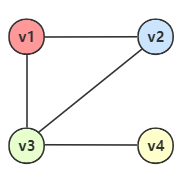{ loading=lazy } 这个图的边集可以变成下面的矩阵：

$\begin{bmatrix} 0 & 1 & 1 & 0 \\\\ 1 & 0 & 1 & 0 \\\\ 1 & 1 & 0 & 1 \\\\ 0 & 0 & 1 & 0 \end{bmatrix}$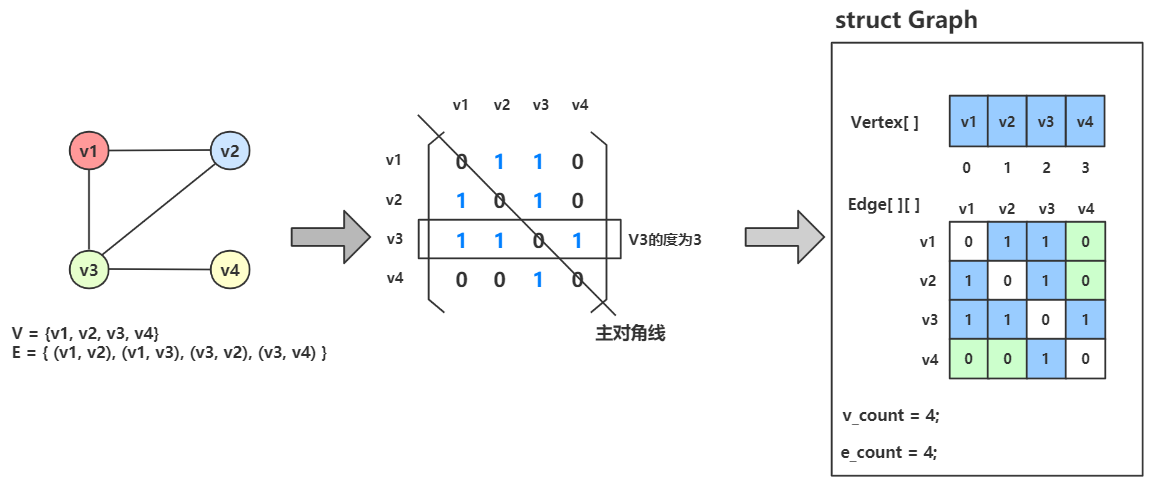Vertex[] 用来存储顶点信息，Edge[][] 用来存储边的信息。

1. 某个顶点的度，起始就是这个顶点 vi 在邻接矩阵中第 i 行（或第 i 列）的元素之和。

eg：顶点 $$v_3$$ 的度就是 1+1+0+1 = 3

2. 求顶点 vi 的所有邻接点就是在邻接矩阵中第 i 行所有元素为 1 的点。

eg：顶点 $$v_2$$ 的所有邻接点就遍历 Edge[...]，其中为 Eege、Edge 为1，表示 $$v_1$$$$v_3$$ 为邻接点。

##### 代码实现¶
  1 2 3 4 5 6 7 8 9 10 11 12 13 14 15 16 17 18 19 20 21 22 23 24 25 26 // GraphModel.h #ifndef GRAPH_MODEL_H_INCLUDED #define GRAPH_MODEL_H_INCLUDED #include #include #include #define MAX 4 typedef enum { ERROR, OK, } Status; typedef enum { DG, // 有向图 UDG, // 无向图 DN, // 有向网 UDN, // 无向网 } GraphKind; typedef char* Vertex; // 顶点数组类型 typedef int Edge; // 邻接矩阵类型 #endif // GRAPH_MODEL_H_INCLUDED 
  1 2 3 4 5 6 7 8 9 10 11 12 13 14 15 16 17 18 19 20 21 22 23 24 25 26 27 28 29 30 // MatrixGraph.h #ifndef MATRIX_GRAPH_H_INCLUDED #define MATRIX_GRAPH_H_INCLUDED #include "GraphModel.h" typedef struct { Vertex vers[MAX]; // 顶点数组 Edge edges[MAX][MAX]; // 邻接矩阵（边数组） size_t v_count; // 顶点总数 size_t e_count; // 边总数 GraphKind kind; // 图类型 } MatrixGraph; /** * @description: 创建无向图 * @param G 要操作初始化的图 * @return 成功返回OK，失败返回ERROR */ Status CreatUDG(MatrixGraph* G); /** * @description: 返回某个顶点在顶点集合中的下标 * @param G 要查找的图结构 * @param v 顶点 * @return 返回下标，不存在返回-1 */ int LocateVex(MatrixGraph* G, Vertex v); #endif // MATRIX_GRAPH_H_INCLUDED 
  1 2 3 4 5 6 7 8 9 10 11 12 13 14 15 16 17 18 19 20 21 22 23 24 25 26 27 28 29 30 31 32 33 34 35 36 37 38 39 40 41 42 43 44 45 46 47 48 49 50 51 52 53 54 55 56 57 58 59 60 61 62 63 64 65 66 67 68 69 70 71 72 73 74 75 76 77 78 79 80 81 82 83 84 85 86 87 88 89 90 91 // MatrixGraph.c #include "MatrixGraph.h" Status CreatUDG(MatrixGraph* G) { G->kind = UDG; printf("Please type the count of Vertex : "); scanf("%d", &G->v_count); printf("Please type the count of Edge : "); scanf("%d", &G->e_count); // 录入顶点数组 printf("Please type Vertex in turn.\n"); for (int i = 0; i < G->v_count; i++) { G->vers[i] = calloc(10, sizeof(Vertex)); printf("Vertex %d :", i); scanf("%s", G->vers[i]); } // 初始化邻接矩阵，所有边的权值设置为0 for (int i = 0; i < G->v_count; i++) { for (int j = 0; j < G->v_count; j++) { G->edges[i][j] = 0; } } // 录入邻接矩阵 printf("Please type Vertex and Adjacent. \n"); for (int i = 0; i < G->e_count; i++) { Vertex v1 = calloc(10, sizeof(Vertex)); Vertex v2 = calloc(10, sizeof(Vertex)); printf("Vertex %d : ", i + 1); scanf("%s", v1); printf("Adjacent %d : ", i + 1); scanf("%s", v2); /** 核心代码 begin */ // 获取顶点的下标 int x = LocateVex(G, v1); int y = LocateVex(G, v2); if (x == -1 || y == -1) return ERROR; // 对应位置置为1 G->edges[x][y] = 1; G->edges[y][x] = 1; /** 核心代码 end */ free(v1); free(v2); } return OK; } int LocateVex(MatrixGraph* G, Vertex v) { int index = 0; while (index < G->v_count) { if (strcmp(v, G->vers[index]) == 0) { break; } index++; } return index == G->v_count ? -1 : index; } void Test() { MatrixGraph G; Status status = CreatUDG(&G); if (status = ERROR) { printf("Create Graph Failed~"); return; } printf("The Adjacent Matrix: \n"); printf("\t"); for (int i = 0; i < G.v_count; i++) { printf("\t%s", G.vers[i]); } printf("\n"); for (int i = 0; i < G.e_count; i++) { printf("\t%s", G.vers[i]); for (int j = 0; j < G.e_count; j++) { printf("\t%d", G.edges[i][j]); } printf("\n"); } } 
 1 2 3 4 5 6 7 #include "MatrixGraph.c" int main(int argc, char const* argv[]) { Test(); return 0; } 

#### 有向图¶

##### 理论描述¶

• $$i$$ 行含义：以结点 $$v_i$$ 为尾的弧（即出度边）
• $$i$$ 列含义：以结点 $$v_i$$ 为头的弧（即入度边）

• 无向图的邻接矩阵一定是对称的，当有向图的邻接矩阵不一定是对称的。
• 顶点 $$v_i$$ 的出度 = 第 $$i$$ 元素之和
• 顶点 $$v_i$$ 的入度 = 第 $$i$$ 元素之和
• 顶点 $$v_i$$ 的度 = 第 $$i$$ 行元素之和 + 第 $$i$$ 列元素之和

Note

• Arc[行][列]
• Arc[出][入]
• 列头入，行尾出：即 列为弧头、入度；行为弧尾、出度。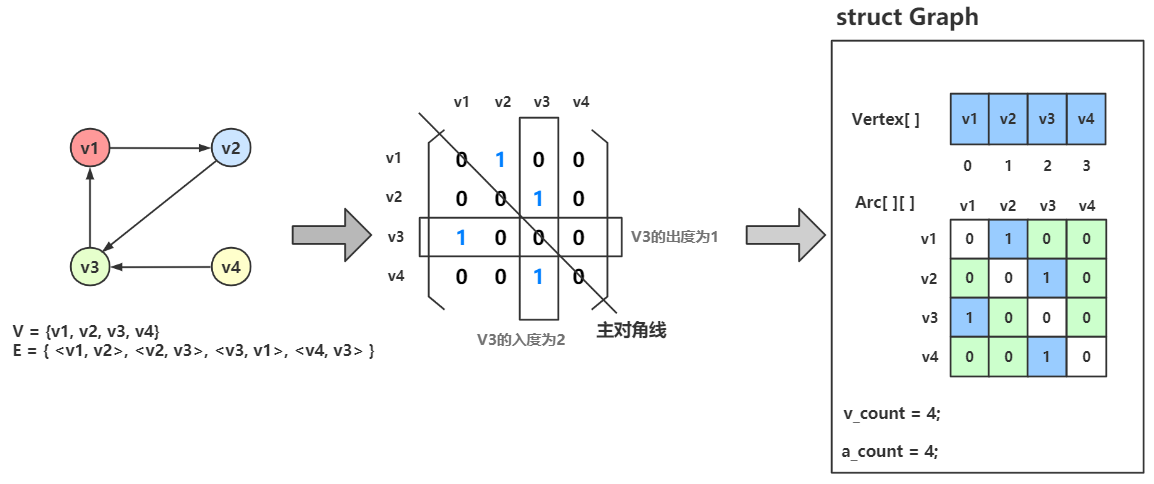##### 代码实现¶

 1 2 G->edges[x][y] = 1; // G->edges[y][x] = 1; 

  1 2 3 4 5 6 7 8 9 10 11 12 13 14 15 16 17 18 19 20 21 22 23 24 25 26 27 28 29 30 31 32 33 34 35 36 Status CreatGraph(MatrixGraph* G, GraphKind kind) { G->kind = kind; // 录入顶点数和边数 ... // 录入顶点数组 ... // 初始化邻接矩阵，所有边的权值设置为0 ... // 录入邻接矩阵 printf("Please type %s and %s. \n", kind == UDG ? "Vertex" : "InDegree", kind == UDG ? "Adjacent" : "OutDegree"); for (int i = 0; i < G->e_count; i++) { Vertex v1 = calloc(10, sizeof(Vertex)); Vertex v2 = calloc(10, sizeof(Vertex)); printf("%s %d : ", kind == UDG ? "Vertex" : "OutDegree", i + 1); scanf("%s", v1); printf("%s %d : ", kind == UDG ? "Adjacent" : "InDegree", i + 1); scanf("%s", v2); int x = LocateVex(G, v1); int y = LocateVex(G, v2); if (x == -1 || y == -1) return ERROR; G->edges[x][y] = 1; G->edges[y][x] = kind == UDG ? 1 : 0; // 无向图置为1，有向图保持默认 free(v1); free(v2); } return OK; } // Status status = CreatGraph(&G); Status status = CreatGraph(&G, DG); 

  1 2 3 4 5 6 7 8 9 10 11 12 13 14 15 16 17 18 19 /** * @description: 获取某个顶点的度 * @param G 要查找的图 * @param v 要查询度的顶点 * @return 该顶点的度 */ size_t GetDegree(MatrixGraph* G, Vertex v) { // 获取无向图的度：累加第i行或第i列的元素之和 // 获取有向图的度：累加第i行和第i列的元素之和并相加 int index = LocateVex(G, v); int inDegree = 0; int outDegree = 0; for (int i = 0; i < G->e_count; i++) { inDegree += G->edges[i][index]; outDegree += G->edges[index][i]; } return G->kind == UDG ? inDegree : inDegree + outDegree; } 

#### 网¶

##### 理论描述¶

$Arc[i][j] = \begin{cases} W_{ij}, & {(v_i, v_j) \in E 或 \langle v_i, v_j \rangle \in E} \\\\ 0, & {i=j} \\\\ ∞, & {反之} \end{cases}$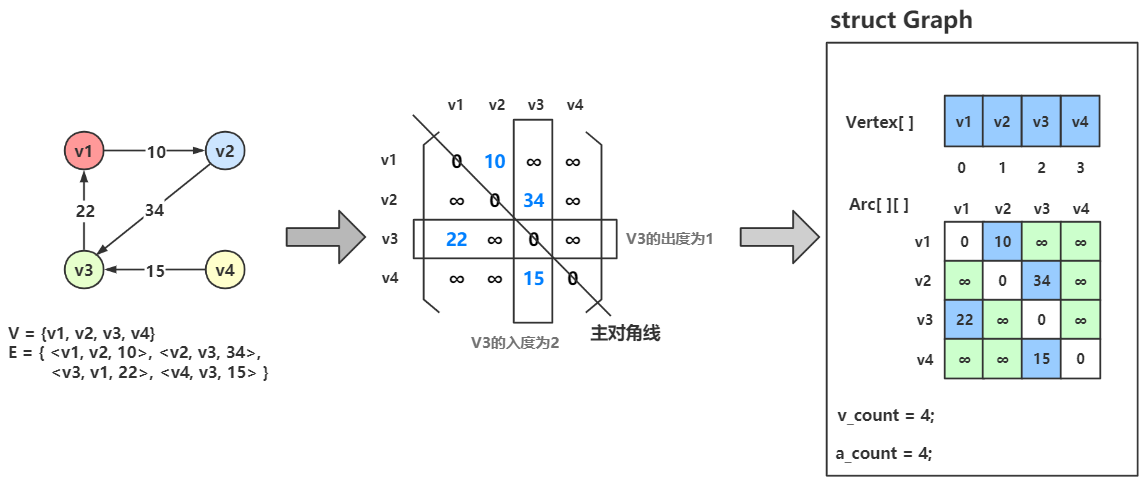##### 代码实现¶

 1 2 3 4 5 6  // 初始化邻接矩阵，所有边的权值设置为无穷 for (int i = 0; i < G->v_count; i++) { for (int j = 0; j < G->v_count; j++) { G->edges[i][j] = INT_MAX; } } 

  1 2 3 4 5 6 7 8 9 10 11 12 13 14 15 16 17 18 19 20 21 22 23 24 25 // 录入邻接矩阵 printf("Please type %s and %s. \n", kind == UDN ? "Vertex" : "InDegree", kind == UDN ? "Adjacent" : "OutDegree"); for (int i = 0; i < G->e_count; i++) { Vertex v1 = calloc(10, sizeof(Vertex)); Vertex v2 = calloc(10, sizeof(Vertex)); int weight = 0; // 权值 printf("%s %d : ", kind == UDN ? "Vertex" : "OutDegree", i + 1); scanf("%s", v1); printf("%s %d : ", kind == UDN ? "Adjacent" : "InDegree", i + 1); scanf("%s", v2); printf("Value %d: ", i + 1); scanf("%s", &weight); int x = LocateVex(G, v1); int y = LocateVex(G, v2); if (x == -1 || y == -1) return ERROR; G->edges[x][y] = weight; G->edges[y][x] = kind == UDN ? weight : INT_MAX; free(v1); free(v2); } 

 1 2 3 4 5 6 7 8  // 邻接矩阵 for (int i = 0; i < G.e_count; i++) { printf("\t%s", G.vers[i]); for (int j = 0; j < G.e_count; j++) { printf("\t%s", G.edges[i][j] == INT_MAX ? "∞" : (char*)G.edges[i][j]); } printf("\n"); } 

Note

#### 无向图的邻接表¶

• 图的顶点集用一维数组存储，数组元素为 数据域(data) + 首邻接点域(firstEdge)
• 图的边/弧集用单链表存储，链表结点由 邻接点域(adjvex) + 指针域(next) 构成；

首邻接点域 指的是顶点的第一个边链表结点的地址，链表中的 邻接点域 存储的是邻接点的在顶点数组中的下标。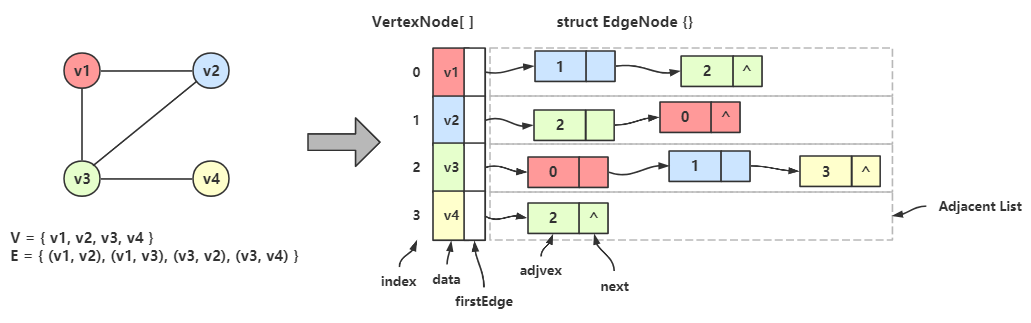eg：$$v_1$$ 的邻接点是 $$v_2$$$$v_3$$，在顶点数组中 $$v_1$$ 的下标是 0；

Vertex 数据域(data) 中存着 $$v_1$$，首邻接点域(firstEdge) 存储着边链的第一个结点的地址；

• 无向图的邻接表重复存储着数据（邻接矩阵里也是），但总比邻接矩阵节省空间。
• 边链中的结点，除了与顶点数组中的元素，相互之间没有关系。
• 顶点的度 = 它的边链的结点数
• 求顶点的所有邻接点 = 遍历顶点的边链并取邻接点域
• 如果有 n 条边，则边链表会有 2n 个结点

#### 有向图的邻接表¶

• 我们以顶点为弧尾来存储边链表，并称这样的边链表为 出边表，或 正邻接表
• 我们以顶点为弧头来存储边链表，并称这样的边链表为 入边表，或 逆邻接表

• 使用入边表表示图时，顶点的入度 = 对应入边表的结点数量，要计算出度只能遍历所有边链表
• 使用出边表表示图时，顶点的出度 = 对应出边表的结点数量，要计算入度只能遍历所有边链表
• 边链表中的结点，除了与顶点元素，相互之间没有关系
• 弧的总数 = 所有边链表的结点的总数之和

#### 有向网的邻接表¶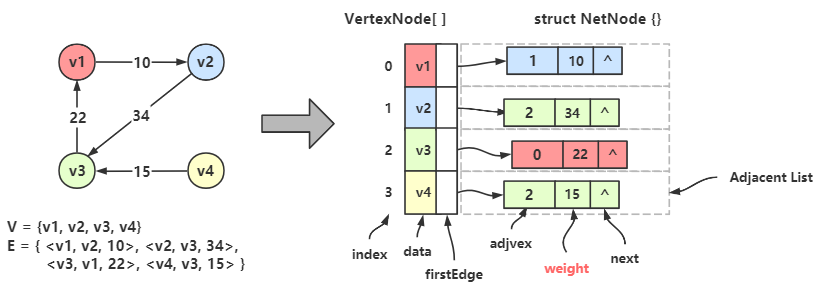#### 代码实现¶

  1 2 3 4 5 6 7 8 9 10 11 12 13 14 15 16 17 18 19 20 21 22 // 边链表结点类型 typedef struct node{ int adjvex; // 邻接点域、邻接点下标 struct node* next; // 指针域 } EdgeNode, ArcNode; // 顶点类型 typedef char* Vertex; // 顶点结点类型 typedef struct { Vertex data; // 数据域 EdgeNode firstEdge; // 首邻接点域 } VertexNode; // 图类型 typedef struct { VertexNode vexs[MAX]; // 顶点结点数组 int v_count; // 顶点数量 int e_count; // 边/弧数量 GraphKind kind; // 图类型 } AdjListGraph; 

### 十字链表 Orthogonal List¶

Note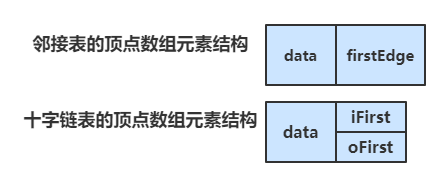data 表示顶点，iFirst 表示入边表的首邻接点域，oFirst 表示出边表的首邻接点域。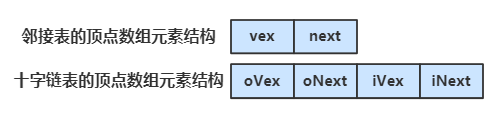oVex 表示弧中出边的结点，即弧尾结点，的下标；oNext 替代了原出边表结点里的指针域；

iVex 表示弧中入边的结点，即弧头结点，的下标；iNext 替代了原入边表结点里的指针域；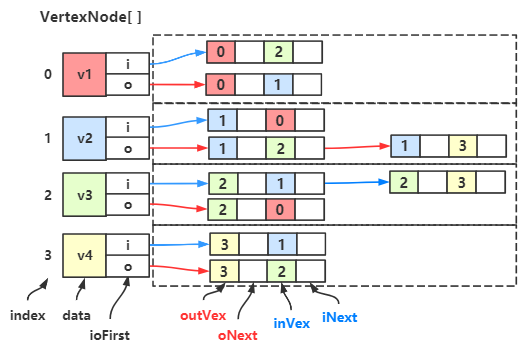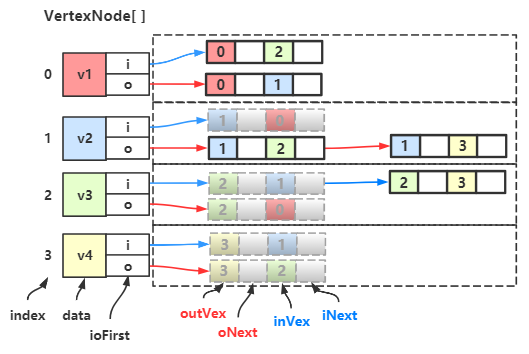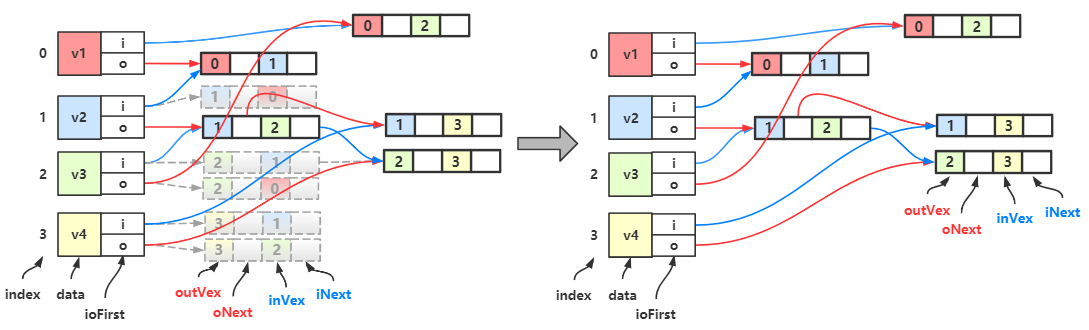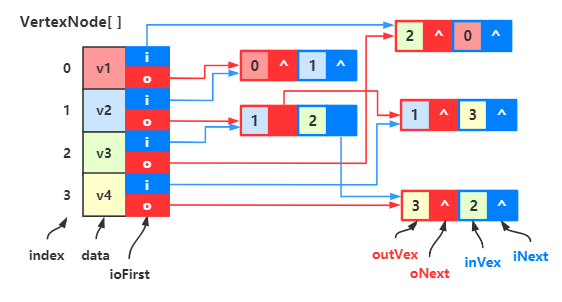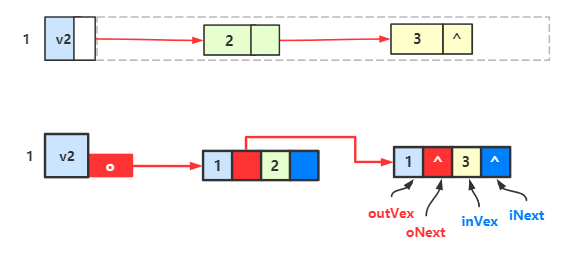#### 代码实现¶

  1 2 3 4 5 6 7 8 9 10 11 12 13 14 15 16 17 18 19 20 21 22 23 24 25 26 /** 顶点类型 */ typedef char* Vertex; /** 十字链表结点 */ typedef struct node{ Vertex oVex; // 弧头下标 Vertex iVex; // 弧尾下标 struct node* oNext; // 出边表指针域 struct node* iNext; // 入边表指针域 int weight; // 权值 } OrthoNode; /** 顶点数组元素类型 */ typedef struct { Vertex data; // 顶点 OrthoNode iFirst; // 入边表的首个结点 OrthoNode oFirst; // 出边表的首个节点 } VertexNode; /** 图结构 */ typedef struct { VertexNode vexArray[MAX]; // 顶点数组 int v_count; // 顶点数量 int a_count; // 弧的数量 GraphKind kind; // 图的类型（有向图/有向网） } OrthoGraph; 

#### 总结¶

Note

1. 生成有向图的出边表和入边表
2. 组合成双邻接表
3. 改造链表结点
4. 删除重复结点
5. 连接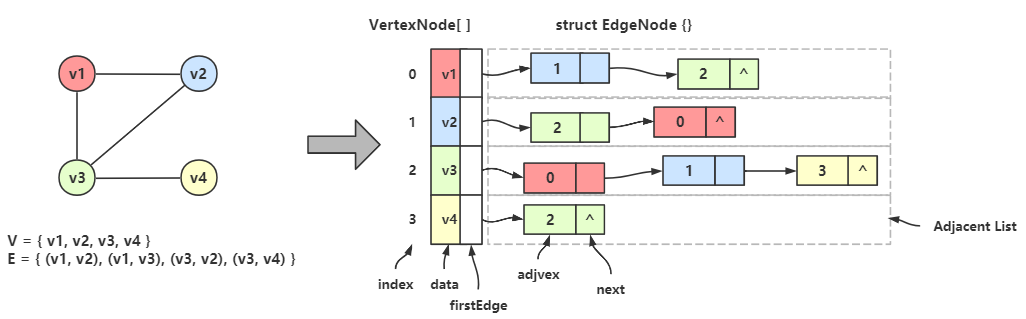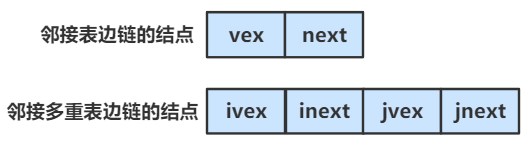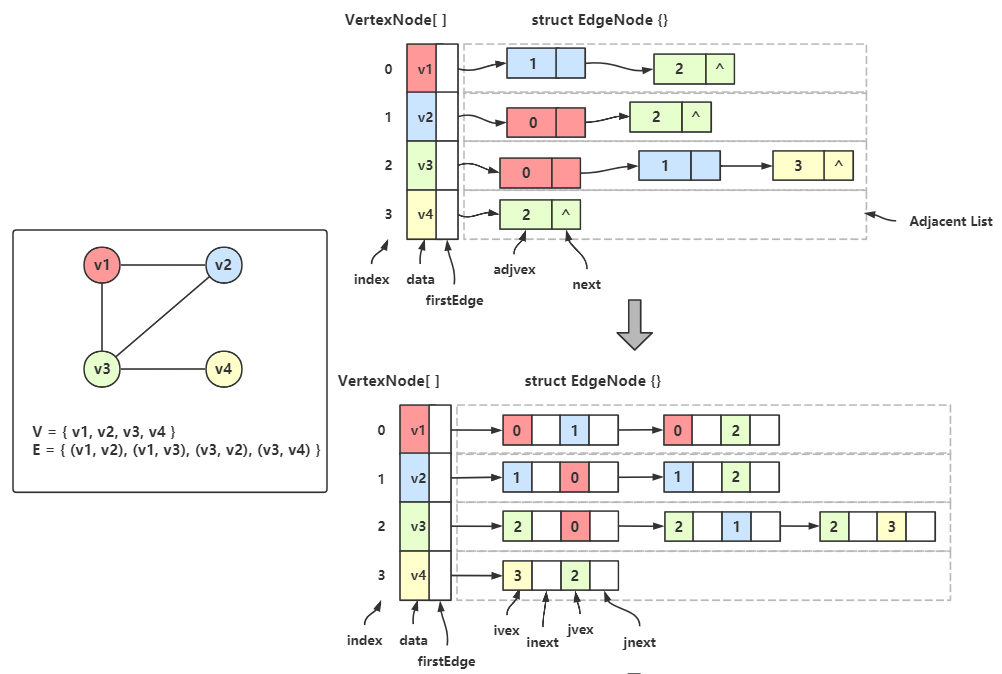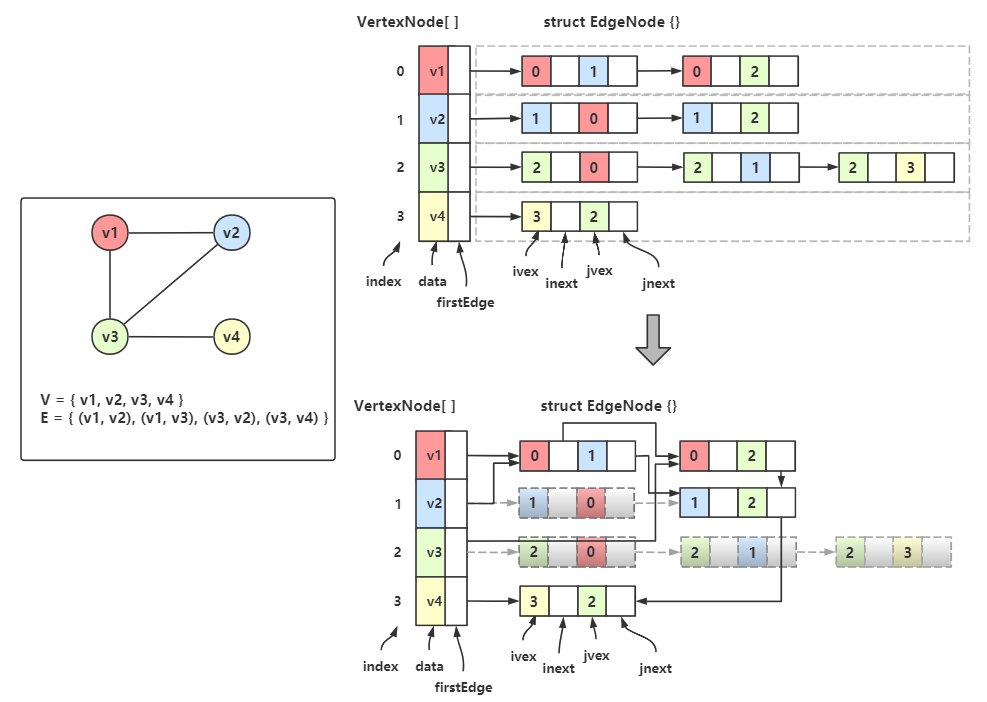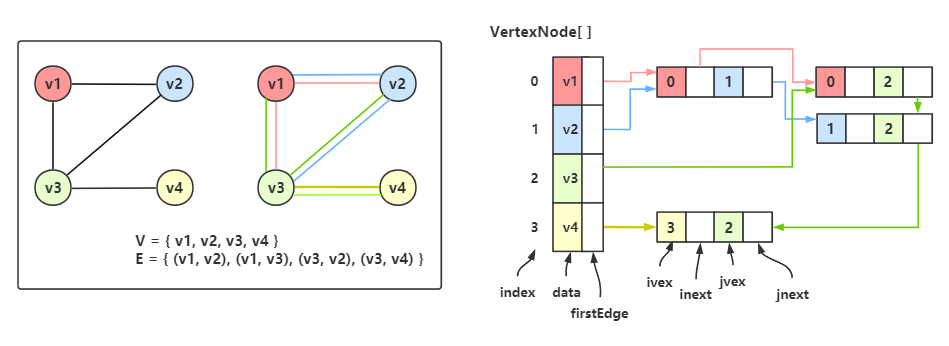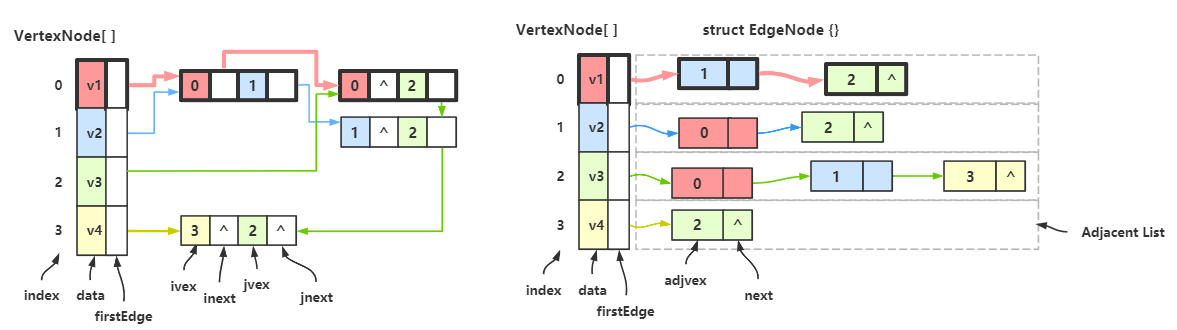Tip

### 边集数组 Edgeset Array¶

Note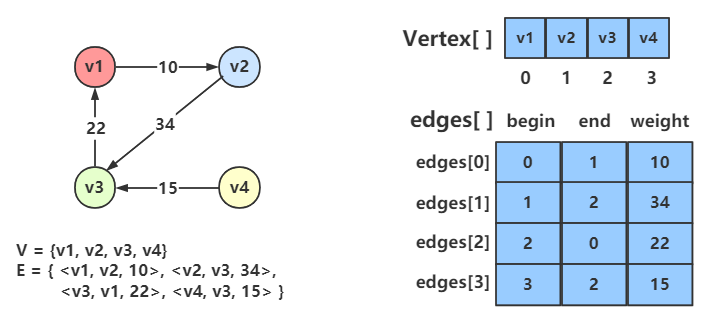### 适用情况总结¶

• 邻接矩阵适合处理稠密图
• 邻接表适合处理稀疏图
• 十字链表适合于需要频繁获取顶点的入度和出度，频繁地判断一个点是不是另一个点的邻接点等的情况
• 邻接多重表主要是针对无向图
• 边集数组适合对边依次进行处理的操作，而不适合对顶点相关的操作

## 图的遍历¶

### 深度优先搜索 DFS¶

DFS 需要借助一个数组来记录访问状态，这里将其称作 状态数组（Status Array）；该数组长度为顶点总数，次序与顶点数组相同。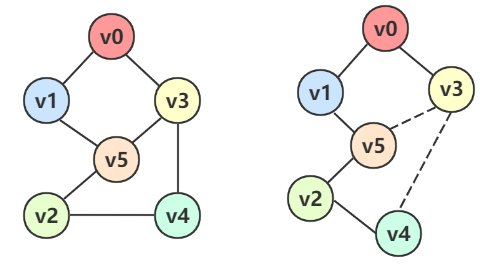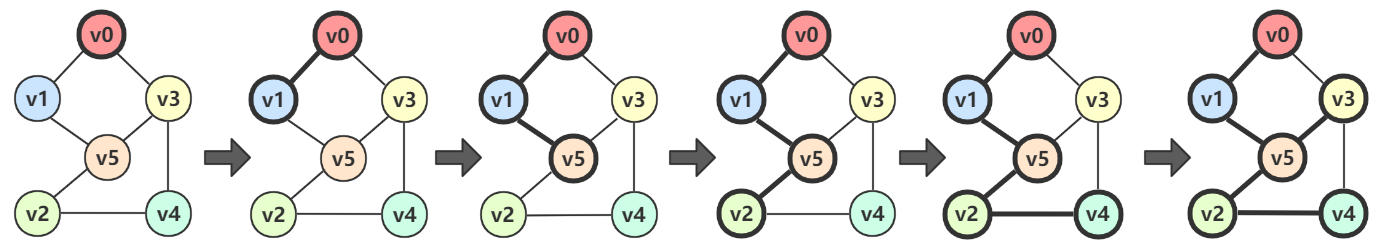• $$v_0$$ 有两个邻接点 $$v_1$$$$v_3$$，我们先选择 $$v_1$$，然后从 $$v_1$$ 出发；
• $$v_1$$ 有两个邻接点 $$v_5$$$$v_0$$$$v_0$$ 已经访问过了，只能选择 $$v_5$$，然后从 $$v_5$$ 出发；
• $$v_5$$ 有三个邻接点 $$v_1$$$$v_2$$$$v_3$$$$v_1$$ 已经访问过了，我们先选择 $$v_2$$ ，然后从 $$v_2$$ 出发；
• $$v_2$$ 有两个邻接点 $$v_5$$$$v_4$$$$v_5$$ 已经访问过了，只能选择 $$v_4$$，然后从 $$v_4$$ 出发；
• $$v_4$$ 有两个邻接点 $$v_2$$$$v_3$$$$v_2$$ 已经访问过了，只能选择 $$v_3$$，然后从 $$v_3$$ 出发；
• $$v_3$$ 有三个邻接点 $$v_4$$$$v_5$$$$v_0$$，全都访问过了，到此遍历也就结束了。

#### 邻接矩阵的 DFS¶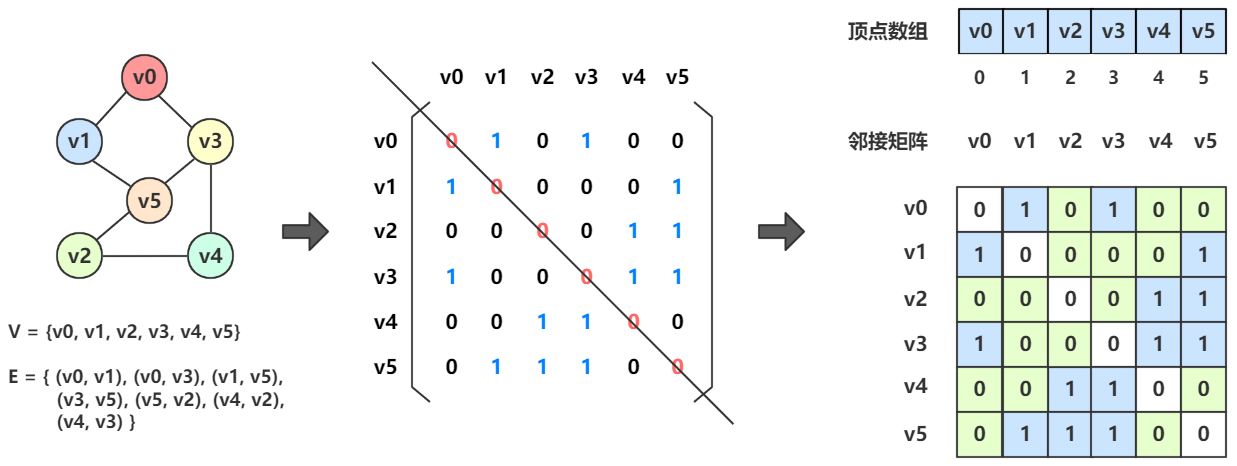• 第一步：先初始化状态数组为未访问状态，即最开始所有顶点都未访问。
• 第二步：遍历顶点数组，让数组里每个顶点都放入遍历执行函数中，不过在调用遍历执行函数之前要先判断顶点是否已被访问。
  1 2 3 4 5 6 7 8 9 10 11 12 13 14 15 16 17 18 19 int visited[MAX]; /** DFS begin */ /** * @description: 深度优先遍历算法 * @param G 需要被遍历的图 * @return 无 */ void DFSTraverse_AMG(MatrixGraph* G) { // 初始化状态数组 for (int i = 0; i < G->v_count; i++) visited[i] = UNVISITED; // DFS 遍历 for (int i = 0; i < G->v_count; i++) if (!visited[i]) // 如果某个顶点未访问 DFS_AMG(G, i); // 调用遍历执行函数 } 

1. 访问当前顶点
2. 更改顶点的访问状态
3. 获取第一个未访问的邻接点下标，然后递归进去，找不到则回溯
  1 2 3 4 5 6 7 8 9 10 11 12 13 14 15 16 /** * @description: 深度优先搜索的核心算法 * @param G 要搜索的图 * @param index 要搜索的顶点的下标 * @return 无 */ void DFS_AMG(MatrixGraph* G, int index) { printf("-> %s ", G->vexes[index]); // 访问当前顶点 visited[index] = VISITED; // 更改当前顶点的访问状态 for (int j = 0; j < G->v_count; j++) if (G->matrix[index][j] && !visited[j]) // 获取第一个未访问的邻接点下标然后递归进去 DFS_AMG(G, j); } /** DFS end */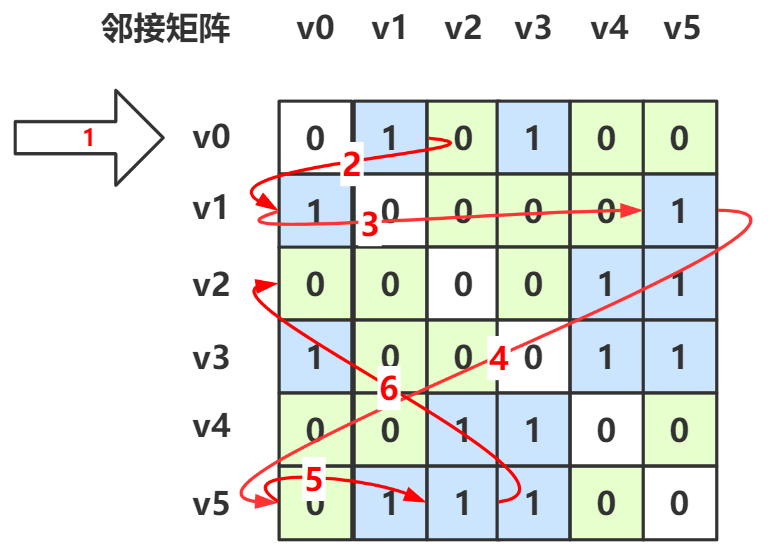• 最开始从第 0 行进行（箭头1），找到第一个邻接点的下标，其列标为 1，于是跳到第 1 行（箭头2）
• 第 1 行第一个邻接点是 v0 ，已经访问过，所以跳过（箭头3），一直找到 $$v_5$$ 这个邻接点，其列标为 5，于是跳到第 5 行（箭头4）
• 第 5 行第一个邻接点的 $$v_1$$，已经访问过，所以跳过（箭头5），接下去找到第二个邻接点 $$v_2$$，其列标为 2，于是跳到第 2 行（箭头6）
• 接下去的步骤都是这个思路，画出来太乱就没画了。

#### 邻接表的 DFS¶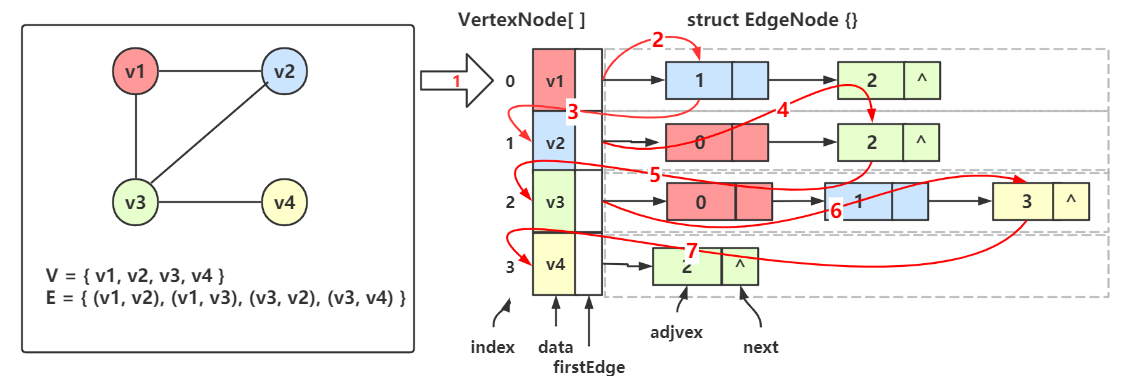• 最开始从 $$v_1$$ 开始（箭头1），访问 $$v_1$$ 的边链上第一个结点（箭头2），得到了 $$v_2$$ 的下标，于是跳到 $$v_2$$（箭头3）
• 接着从 $$v_2$$ 开始，先访问 $$v_2$$，然后遍历 $$v_2$$ 的边链上的结点（箭头4），第一个结点 0 是 $$v_1$$ 的下标，已经访问过了，于是继续下一个结点，得到了 $$v_3$$ 的下标，于是跳到 $$v_3$$（箭头5）
• 接着从 $$v_3$$ 开始，先访问 $$v_3$$，然后遍历 $$v_3$$ 的边链上的结点（箭头6），第一和第二个结点是 $$v_1$$$$v_2$$ 的下标，已经访问过，所以接着下一个结点，得到 $$v_4$$ 的下标，于是跳到 $$v_4$$（箭头7）
• 接着从 $$v_4$$ 开始，先访问 $$v_4$$，然后遍历 $$v_4$$ 的边链上的结点，发现得到的是 $$v_3$$ 的下标，已经访问过了，再下去没有了，于是结束。

  1 2 3 4 5 6 7 8 9 10 11 12 13 14 15 16 17 18 19 20 21 22 23 24 void DFSTraverse_ALG(AdjListGraph* G) { // 初始化状态数组 for (int i = 0; i < G->v_count; i++) visited[i] = UNVISITED; // DFS遍历 for (int i = 0; i < G->v_count; i++) if (!visited[i]) // 如果顶点没有被访问，就递归调用 DFS_ALG(G, i); } void DFS_ALG(AdjListGraph* G, int index) { printf(" -> %s", G->vexes[index].data); // 访问顶点 visited[index] = VISITED; // 更改状态 // 寻找邻接点 EdgeNode* eNode = G->vexes[index].firstEdge; while (eNode) { if (!visited[eNode->adjvex]) // 如果邻接点未访问就递归访问 DFS_ALG(G, eNode->adjvex); eNode = eNode->nextEdge; } } 
DFSTraverse_ALG()DFSTraverse_AMG() 是一样的，初始化状态数组，然后遍历顶点数组，调用 DFS 算法

DFS_AMG() 中是遍历某一行，DFS_ALG() 中是遍历某一条链表，执行的逻辑都是如果邻接点为访问就递归。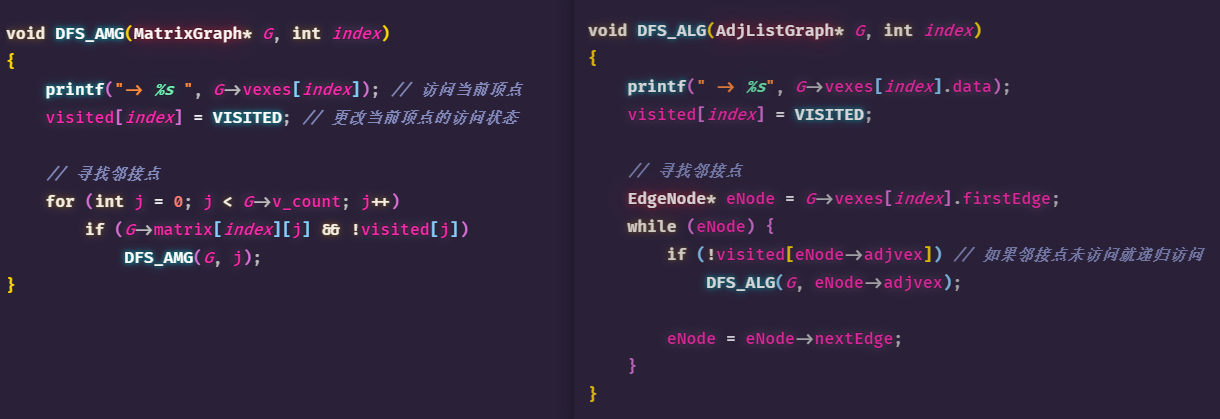#### 时间复杂度¶

• 当用邻接矩阵时，使用的是二位数组，查找每个顶点的邻接点所需时间为 $$O(n^2)$$，n 为图中顶点数。
• 当用邻接表时，使用的是链表，查找每个顶点的邻接点所需时间为 $$O(e)$$，e 为无向图中边的数量，或有向图中弧的数量。

### 广度优先 BFS¶

BFS 的思想是，把顶点都放到队列中，当访问一个顶点，即为出队一个元素；当出队一个元素时，需将其所有未访问的邻接点入队。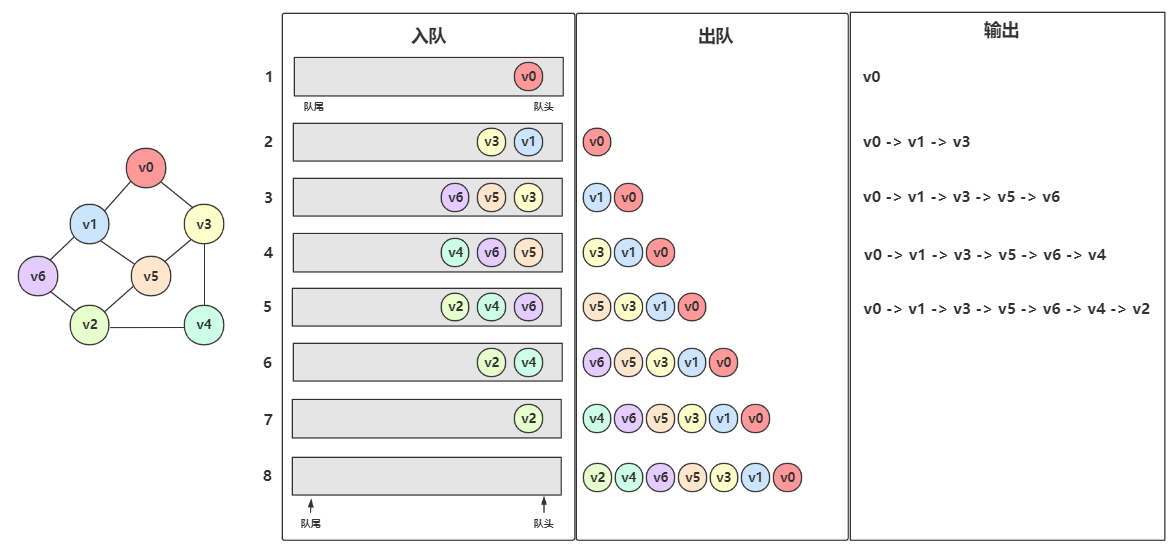1. 先从 $$v_0$$ 开始遍历，打印 $$v_0$$，然后 $$v_0$$ 入队
2. 队列不为空，$$v_0$$ 出队，同时 $$v_0$$ 的未入队且未访问的邻接点 $$v_1$$$$v_3$$ 入队，然后访问 $$v_1$$$$v_3$$
3. 队列不为空，$$v_1$$ 出队，同时 $$v_1$$ 的未入队且未访问的邻接点 $$v_5$$$$v_6$$ 入队，然后访问 $$v_5$$$$v_6$$
4. 队列不为空，$$v_3$$ 出队，同时 $$v_3$$ 的未入队且未访问的邻接点 $$v_4$$ 入队，然后访问 $$v_4$$
5. 队列不为空，$$v_5$$ 出队，同时 $$v_5$$ 的未入队且未访问的邻接点 $$v_2$$ 入队，然后访问 $$v_2$$
6. 队列不为空，$$v_6$$ 出队，同时 $$v_6$$ 已经没有未入队且为访问的邻接点了，不做入队操作
7. 队列不为空，$$v_4$$ 出队
8. 队列不为空，$$v_2$$ 出队

#### 队列¶

BFS 需要借助队列实现，下面是队列的代码：

  1 2 3 4 5 6 7 8 9 10 11 12 13 14 15 16 17 18 19 20 21 22 23 24 25 26 // LinkQueue.h #ifndef LINK_QUEUE_H_INCLUDED #define LINK_QUEUE_H_INCLUDED #include "GraphModel.h" typedef Vertex Element; // 结点 typedef struct QueueNode { Element data; struct QueueNode* next; } QueueNode; // 队列 typedef struct { QueueNode* front; QueueNode* rear; } LinkedQueue; Status InitLinkedQueue(LinkedQueue* q); Status EnQueue(LinkedQueue* q, Element e); Status DeQueue(LinkedQueue* q, Element* e); bool isEmptyQueue(LinkedQueue* q); void PrintLinkedQueue(LinkedQueue* q); #endif // LINK_QUEUE_H_INCLUDED 
  1 2 3 4 5 6 7 8 9 10 11 12 13 14 15 16 17 18 19 20 21 22 23 24 25 26 27 28 29 30 31 32 33 34 35 36 37 38 39 40 41 42 43 44 45 46 47 48 49 50 51 52 53 54 55 56 57 58 59 60 61 62 63 64 65 66 // LinkQueue.c #include "LinkQueue.h" Status InitLinkedQueue(LinkedQueue* q) { q->front = NULL; q->rear = NULL; return OK; } Status EnQueue(LinkedQueue* q, Element e) { QueueNode* new = malloc(sizeof(QueueNode)); new->data = e; new->next = NULL; if (isEmptyQueue(q)) { // 处理空队情况 q->front = q->rear = new; return OK; } else { // 处理非空队情况 q->rear->next = new; // 原队尾结点的next指向新结点 q->rear = new; // 更新队尾指针 return OK; } return ERROR; } Status DeQueue(LinkedQueue* q, Element* e) { if (isEmptyQueue(q)) { // 空队直接返回 e = NULL; return OK; } if (q->front == q->rear) { // 处理队中只有一个结点的情况 *e = q->front->data; free(q->front); q->front = q->rear = NULL; return OK; } // 处理队中不止一个结点的情况 QueueNode* del = q->front; // 标记队头结点 q->front = del->next; // 队头指针指向下一个结点 *e = del->data; // 取数据 free(del); // 删除原队头结点 del = NULL; return OK; } bool isEmptyQueue(LinkedQueue* q) { return q->front == NULL || q->rear == NULL; } void PrintLinkedQueue(LinkedQueue* q) { if (isEmptyQueue(q)) { printf("Queue Empty"); } QueueNode* p = q->front; while (p) { printf("\t%s ", p->data); p = p->next; } } 

#### 邻接矩阵的 BFS¶

  1 2 3 4 5 6 7 8 9 10 11 12 13 14 15 16 17 18 19 20 21 22 23 24 25 26 27 28 29 30 31 32 33 34 35 36 37 38 39 40 41 42 43 44 45 46 47 48 49 50 51 52 53 54 55 56 57 58 59 60 61 62 63 64 65 66 67 /** BFS begin */ void BFSTraverse_AMG(MatrixGraph* G) { for (int i = 0; i < G->v_count; i++) visited[i] = UNVISITED; // 循环遍历每个顶点 for (int i = 0; i < G->v_count; i++) if (!visited[i]) // 如果没有访问过就遍历访问 BFS_AMG(G, i); } void BFS_AMG(MatrixGraph* G, int index) { printf("-> %s ", G->vexes[index]); // 访问顶点 visited[index] = VISITED; // 更改访问状态 LinkQueue queue; InitLinkedQueue(&queue); // 初始化队列 EnQueue(&queue, G->vexes[index]); // 当前顶点入队，对应上图中第一步的 v0 入队 while (!isEmptyQueue(&queue)) { // 取出队头元素，遍历队头顶点的所有邻接点 Vertex vex; DeQueue(&queue, &vex); // 取出的队头顶点 // 获取该顶点的所有邻接点 for (int i = FirstAdjVex_AMG(G, vex); i; i = SecondAdjVex_AMG(G, vex, G->vexes[i])) { if (!visited[i]) { printf("-> %s ", G->vexes[i]); // 遇到顶点的邻接点先访问 visited[i] = VISITED; EnQueue(&queue, G->vexes[i]); // 再入队 } } } } int FirstAdjVex_AMG(MatrixGraph* G, Vertex v) { int defaultWeight = G->kind <= 1 ? 0 : INT_MAX; // 图/网 的默认权重 int vex_index = LocateVex(G, v); // 获取顶点下标 if (vex_index == -1) return ERROR; // 搜索图的邻接矩阵中域顶点v的第一个邻接点下标 for (int j = 0; j < G->v_count; j++) if (G->matrix[vex_index][j] != defaultWeight) return j; return 0; } int SecondAdjVex_AMG(MatrixGraph* G, Vertex v1, Vertex v2) { int defaultWeight = G->kind <= 1 ? 0 : INT_MAX; // 图/网 的默认权重 int index1 = LocateVex(G, v1); int index2 = LocateVex(G, v2); if (index1 == -1 || index2 == -1) return 0; for (int i = index2 + 1; i < G->v_count; i++) if (G->matrix[index1][i] != defaultWeight) return i; return 0; } /** BFS end */ 

#### 邻接表的 BFS¶

  1 2 3 4 5 6 7 8 9 10 11 12 13 14 15 16 17 18 19 20 21 22 23 24 25 26 27 28 29 30 31 32 33 34 void BFSTraverse_ALG(AdjListGraph* G) { for (int i = 0; i < G->v_count; i++) // 初始化状态数组 visited[i] = UNVISITED; for (int i = 0; i < G->v_count; i++) // 遍历顶点数组，逐一对每一个未访问的顶点执行 BFS if (!visited[i]) BFS_ALG(G, i); } void BFS_ALG(AdjListGraph* G, int index) { printf("-> %s ", G->vexes[index].data); // 访问顶点 visited[index] = VISITED; // 更改顶点状态 LinkQueue queue; InitLinkedQueue(&queue); EnQueue(&queue, G->vexes[index].data); // 当前顶点入队 while (!isEmptyQueue(&queue)) { // 取出队头元素，遍历队头顶点的所有邻接点 Vertex vex; DeQueue(&queue, &vex); // 取出队头元素 // 遍历所有邻接点 EdgeNode* node = G->vexes[LocateVex_ADJ(G, vex)].firstEdge; while (node) { if (!visited[node->adjvex]) { printf("-> %s ", G->vexes[node->adjvex].data); // 邻接点先访问 visited[node->adjvex] = VISITED; EnQueue(&queue, G->vexes[node->adjvex].data); // 再入队 } node = node->nextEdge; } } } 

## 总结¶

1. 存储结构：

• 邻接矩阵：一维数组存储顶点集+二维数组存储边/弧
• 邻接表：一维数组存储顶点集+单链表存储边/弧
• 出边表：链表的结点存储的是弧头的下标
• 入边表：链表的结点存储的是弧尾的下标
• 十字链表：将出边表和入边表整合起来，主要针对有向图
• 邻接多重表：将所有边链的结点整合起来，去除重复的结点，主要针对无向图
• 边集数组：一维数组存储顶点集+一维数组存储边集，关注的是边
2. 图的遍历：

• 深度优先搜索 DFS广度优先搜索 BFS 是图的最基本的遍历方式。
• DFS 是仿树的前序遍历，利用栈做辅助，具体实现是不断将当前顶点的第一个未访问邻接点拿去递归。
• BFS 是仿树的层序遍历，利用队列做辅助，具体实现是每当出队一个元素时，就访问其所有未访问且未入队的邻接点，并将这些邻接点入队。
• 使用邻接矩阵存储和使用邻接表存储的图在做 DFS 或 BFS 时没什么区别，只不过邻接矩阵是操作二维数组，邻接表是操作链表。

1. 对称矩阵：n 阶矩阵的元满足 $$a_{ij} = a_{ji}, (0 \leq i, j \leq n)$$，即从矩阵左上角到右下角的主对角线为轴，右上角的元与左下角相对应的元完全相等。